Faraday's Law MCQ Level - 2

# Faraday's Law MCQ Level - 2

Test Description

## 10 Questions MCQ Test Topic wise Tests for IIT JAM Physics | Faraday's Law MCQ Level - 2

Faraday's Law MCQ Level - 2 for Physics 2023 is part of Topic wise Tests for IIT JAM Physics preparation. The Faraday's Law MCQ Level - 2 questions and answers have been prepared according to the Physics exam syllabus.The Faraday's Law MCQ Level - 2 MCQs are made for Physics 2023 Exam. Find important definitions, questions, notes, meanings, examples, exercises, MCQs and online tests for Faraday's Law MCQ Level - 2 below.
Solutions of Faraday's Law MCQ Level - 2 questions in English are available as part of our Topic wise Tests for IIT JAM Physics for Physics & Faraday's Law MCQ Level - 2 solutions in Hindi for Topic wise Tests for IIT JAM Physics course. Download more important topics, notes, lectures and mock test series for Physics Exam by signing up for free. Attempt Faraday's Law MCQ Level - 2 | 10 questions in 45 minutes | Mock test for Physics preparation | Free important questions MCQ to study Topic wise Tests for IIT JAM Physics for Physics Exam | Download free PDF with solutions
 1 Crore+ students have signed up on EduRev. Have you?
Faraday's Law MCQ Level - 2 - Question 1

### The current in inner coil is i = 2t2, as shown in figure. The current induced in the outer coil has a resistance of R.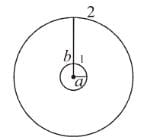Detailed Solution for Faraday's Law MCQ Level - 2 - Question 1

Let I  be the current be in the outer coil 2.
The field at centre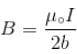The flux through the inner coil due to current in the outer coil 2,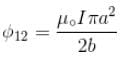So, Mutual inductance of outer coil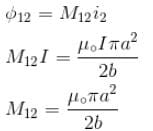Flux across the outer coil,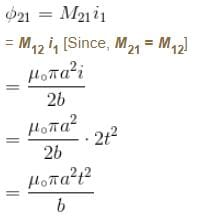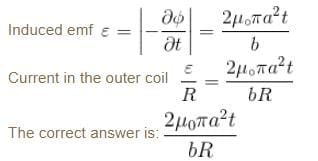Faraday's Law MCQ Level - 2 - Question 2

### A circular loop of radius a is made of a single turn of thin conducting wire. The self inductance of this loop is L. If the number of turns in the loop is increased from 1 to 8, the self inductance would be :

Detailed Solution for Faraday's Law MCQ Level - 2 - Question 2

Let us consider there are n  turns of a coil having radius a and current  I. Then magnetic field at the centre of the coil is given by.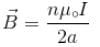Flux of magnetic field through a coil.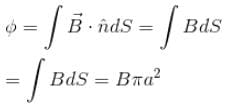Flux through n  coil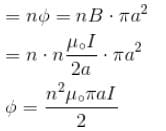Self inductance given by relation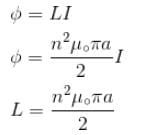If number of turns in the loop is increased from 1 to 8 then, Self inductance increased by 64 time.

Faraday's Law MCQ Level - 2 - Question 3

### A large circular coil of N  turns and radius R carries a time varying current I = I0 sin (ωt). A small circular coil of n  turns and radius r (r << R) is placed at the center of the large coil such that the coils are concentric and coplanar. The induced emf in the small coil :

Detailed Solution for Faraday's Law MCQ Level - 2 - Question 3

Flux through small coil is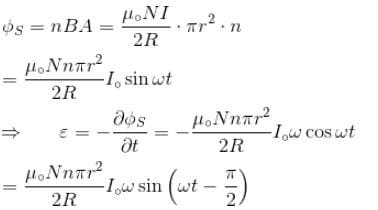Hence, emf  is lay behind the large coil current by π/2
The correct answer is: lags the current in the large coil by π/2

Faraday's Law MCQ Level - 2 - Question 4

A metal disc of radius a  rotates with an angular velocity ω about an axis perpendicular to its plane passing through its centre in a magnetic field of induction B  acting perpendicular to the plane of the disc. The induced emf between the rim and axis of the disc.

Detailed Solution for Faraday's Law MCQ Level - 2 - Question 4

In the presence of uniform magnetic field B perpendicular to the disc in outward direction (say) Force on a unit positive charge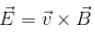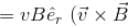will lie in radially outward direction)
Work done in moving a unit positive charge from centre to the rim is equal to potential difference ε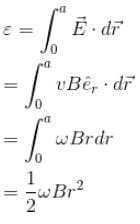The correct answer is: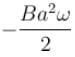Faraday's Law MCQ Level - 2 - Question 5

A wire coil of length l, mass m, is sliding without friction on conducting rail ax and by as shown in figure. The vertical rails are connected to one another via an external resistance  R. The entire circuit is placed in a region of space having a uniform magnetic field B. The field is perpendicular to the plane of circuit and directed outwards. The steady speed of rod cd  is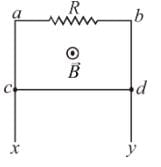Detailed Solution for Faraday's Law MCQ Level - 2 - Question 5

Let wire cd  is at distance x  from the edge be considered a plane surface bounded by the loop acdb  and unit normal vector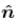lying in same direction as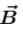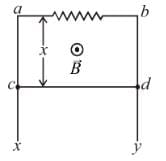Flux through area  acdb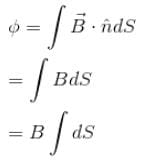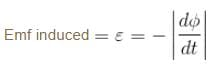Blv
Since, Flux is increasing with time, emf will be induced so as to circulate current resulting in weakening of magnetic field. So, current should flow from P  to  Q.
Current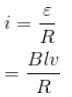Lorentz force acting on rod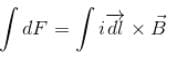ilB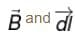are perpendicular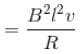(forward up the incline)

Free body diagram of slide is shown in figure.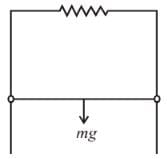There is no acceleration in direction perpendicular to plane of the loop.

So, N = O   [N  Normal reaction perpendicular to the plane]

But in the plane of the loop, the wire cd  slide down due to gravitational force acting downward and lorentz force acting upward.

So, In downward direction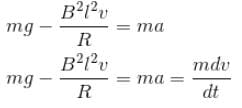But when we talk about steady speed,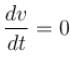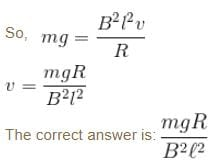Faraday's Law MCQ Level - 2 - Question 6

A loop is formed by two parallel conductor connected by a solenoid with inductance L and a conducting rod of mass m  which can freely (without friction) side over the conductors. The conductors are located in horizontal plane in a uniform vertical magnetic field B. The distance between the conductor is l. Then angular frequency of rod.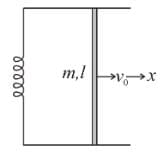Detailed Solution for Faraday's Law MCQ Level - 2 - Question 6

Let at any instant of time, velocity of the rod is  towards right. The current in the circuit is i. In the figure,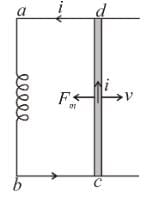Va – Vb = Vd – Vc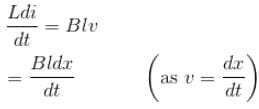i.e. Ldi = Bldx
Li
= Blx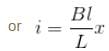Magnetic force on the rod at this instant is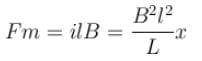Since, this force is in opposite direction of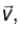so from Newton’s second law we an write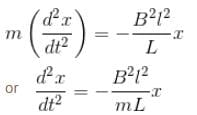Comparing this with equation of SHM, i.e.,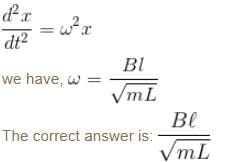Faraday's Law MCQ Level - 2 - Question 7

A conducting rod of length a is rotating with constant angular speed ω about its perpendicular bisector. A uniform magnetic field B exist parallel to the axis of rotation. The emf induced between two ends of the rod is.

Detailed Solution for Faraday's Law MCQ Level - 2 - Question 7

Let us calculate motional emf produced in the rod. Consider a unit positive charge on the rod at a distance r  from the centre. Let rod rotates with angular velocity ω. Velocity of this unit positive charge in magnetic field in transverse direction is ωr.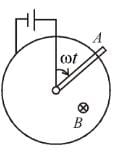Lorentz force acting on the unit charge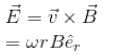Potential difference between A  and  O = VAO

work done in moving a unit positive charge from O  to A.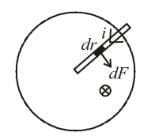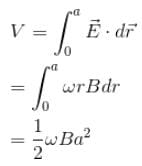The correct answer is: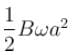Faraday's Law MCQ Level - 2 - Question 8

An infinite long wire carrying a current I(t) = I0 cos(ωt) is placed at a distance a from a square loop of side a  in the figure. If the resistance of the loop is  R, then the amplitude of the induced current in the loop is :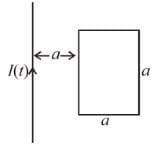Detailed Solution for Faraday's Law MCQ Level - 2 - Question 8

To find out the flux through the loop. Consider an element of length a and breadth dx at a distance x  from wire.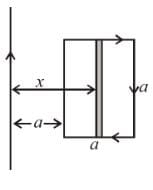∴  Area = adx = dS

Let the orientation of the loop is such that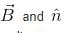lie in same direction,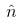is unit normal to surface.

Magnetic field due to long current carrying conductor wire.

at a distance x is given by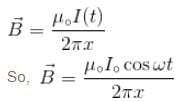Flux of magnetic field through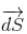element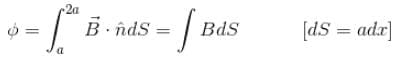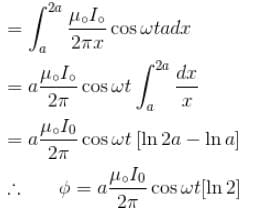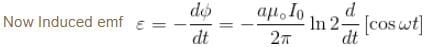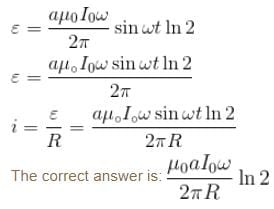Faraday's Law MCQ Level - 2 - Question 9

Two coil of self inductance L1 and L2 are placed closed to each other so that total flux in one coil is completely linked with other. If M is the mutual inductance between them, then :

Detailed Solution for Faraday's Law MCQ Level - 2 - Question 9

When the flux changes in the second coil due to change in the current in first coil, then the mutual inductance is given by the relation.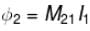Similarly, when flux changes in first coil due to change in the current in second coil.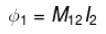From the Reciprocity Theorem
M21 = M12 = M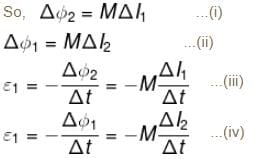From (iii) and (iv)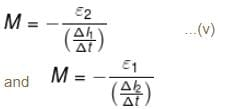Multiply (v) and (vi)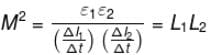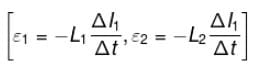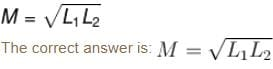Faraday's Law MCQ Level - 2 - Question 10

Two parallel rails separated by a distance l are connected through a resistance R  in yz plane. Magnetic field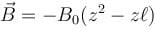is present in the direction perpendicular to the plane of the rails. A conducting rod of mass. m  placed on the rails and given a velocity of v0 parallel to the plane of rails. The expression for the velocity.

Detailed Solution for Faraday's Law MCQ Level - 2 - Question 10

Let the distance of slider from AB is y. Consider an element of length y and width dz  at a distance z from the y axis.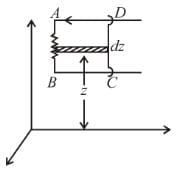dS = ydz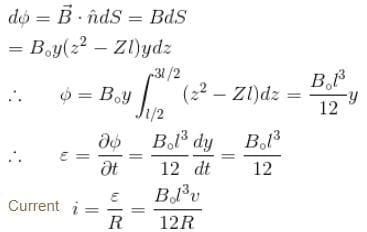consider a length dz of the rod

∴  Magnetic force on rod dF = iB·dz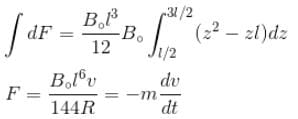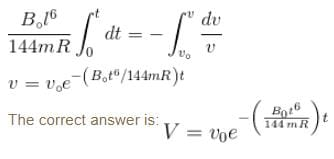## Topic wise Tests for IIT JAM Physics

217 tests
 Use Code STAYHOME200 and get INR 200 additional OFF Use Coupon Code